Panorama’s primary job is storing and retrieving data. The primary job of formulas is to combine and manipulate data, both numeric and textual. Here are some typical tasks that are performed with formulas:
• Automatically add up all the items in an invoice and calculate the sales tax.
• Automatically divide all the names in a database into separate first and last names, or convert all the company names in a database to upper case.
• Automatically look up the price of an item in inventory, or check the quantity on hand.
• Automatically look up and display the items on a customer’s previous order.

As you can see, you’ll need to learn how to use formulas to get the most from your Panorama investment. Fortunately, formulas are easy to learn and use, especially for the most common mathematical formulas like totals, taxes and percentages.

#### Formula Workshop

The Formula Workshop, available in the Help menu, lets you quickly test formulas and perform calculations. It’s great for quick experiments with formulas before you put them into actual use.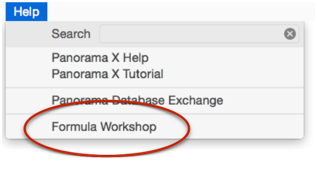Formula results are calculated immediately as you type, there is no need to press Return or Enter.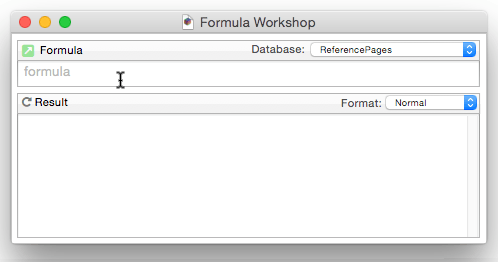To learn more about this workshop, see Formula Workshop.

#### Formula Components

Just as a sentence is constructed from basic words, a formula is created by combining simple elements — values (also called operands), operators and functions. Values (operands) are roughly equivalent to nouns, while operators and functions act as verbs. This illustration show the components that go into a typical formula.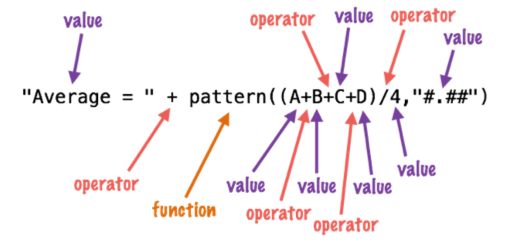#### Formula Grammar

Panorama formulas have grammar rules just as human languages like English and Spanish do. These rules tell how values, operands and functions can be combined to make a valid formula.

The simplest formula is a single data value. Here are four examples of such simple formulas.

``````A
47
"Oregami"
ShippingMethod
``````

Two values can be combined with an operator in between. The first example below adds two numbers together. The second example multiplies two numbers together. The third example appends two text values together (to produce a value like Mr. Jones).

``````2+2
Total * TaxRate
"Mr. " + LastName
``````

The values must be the appropriate type for the operator. For example, you can multiply two numbers together like this

``````2*2
``````

but you cannot multiply two text values together like this (see Grammar Errors below).

``````"Mr. " * LastName
``````

You can combine three or more data values with an operator between each pair of values.

``````7+3*4/2
FirstName + " " + MiddleInitial + " " + LastName
``````

#### Calculation Order and Parentheses

When a formula contains more than one operator, the calculations are performed from left to right unless one of the operators has a higher precedence (priority). This is the natural arithmetic order—multiply and division first, then addition and subtraction. This list shows the order of precedence for all operators.

1. Unary minus (example: -12)
2. Raise to power (example: 10^5)
3. Multiply and Divide
4. Integer Divide
5. MOD operator (remainder)
6. Add and Subtract
7. Comparisons (=, <>, <, >, ...)
8. NOT
9. AND
10. XOR
11. OR

For example, consider the formula below.

``````7+3*4/2
``````

Panorama first multiplies 3 * 4 to get 12, then divides this by 2 to get 6. Finally it adds 7 (addition is last because of its low precedence) to get the final result, 13.

You can override the natural calculation order with parentheses. For example, the parentheses in the formula below force the addition to be calculated first, then the multiplication and division.

``````(7 + 3) * 4 / 2
``````

Now the final result is 20 instead of 13. When in doubt you can always add parentheses to force Panorama to calculate the formula in any order you want.

#### Functions

A function is a formula component that calculates a value. It may calculate the value out of thin air (for example, calculating the value of the current date or time) or it can calculate the value from other values (for example trigonometric functions calculate values from angles). Panorama has several hundred functions available. Each function has a name, and is always followed by parentheses. For example, the tan( function calculates the tangent (a trigonometric function) of an angle.

``````tan(30)
``````

A function can be used in a formula anywhere a regular value can be used. Just as with ordinary values, you can use operators to combine functions with other values (and functions).

``````3 + tan(35)
``````

The value operated on by the function is called a parameter.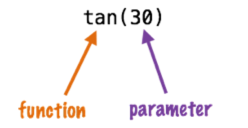A function takes the parameter value (in this case 35) and transforms it into another value (in this case 0.4738, the tangent of 35). The parameter can be a formula itself, like this.

``````tan( A + B )
``````

In this case Panorama first calculates the value A+B, then computes the tangent of that sum. A parameter may be as complex a formula as you need, with additional parentheses and even other functions nested inside the first function.

``````tan( sqr( A + B ) + 1 )
``````

The parameter to the sqr( function is A+B, while the parameter to the tan( function is sqr(A+B)+1. (The sqr( function, by the way, calculates square roots.) Panorama will always calculate the formula from the inside out until the entire formula has been computed.

#### Multi-Parameter Functions

Many functions use more than one parameter. When more than one parameter is required each parameter is separated from the next by a comma. All of the parameters are surrounded by parentheses, just as with single parameter functions. For example, the pattern( function (shown below) requires two parameters. The first parameter must be a numeric value (in this case a calculated average) and the second parameter must be a text value containing a pattern for formatting the number (see Numeric Patterns).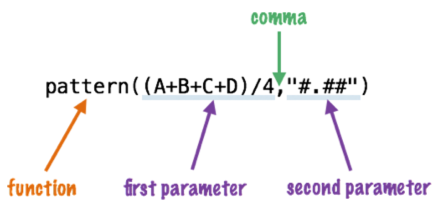Some functions require as many as six parameters.

#### Required vs. Optional Parameters

Most function parameters are required — you must supply them every time you use the function. However, some functions have optional parameters. You can leave these off if you don’t need them. If a function has optional parameters, that will be mentioned in the documentation page for that function.

#### Zero Parameter Functions

A small handful of functions don’t require any parameters at all. These functions generate a value all by themselves, either by consulting the computer hardware (current date, current time), querying internal Panorama data (line number, imported data) or by generating a completely random number each time the formula is computed.

``````today() ☞ current date
now() ☞ current time
seq() ☞ line number
import() ☞ line of text from import file
rnd() ☞ random number
``````

As you can see, these functions have no parameter within the parentheses. You cannot omit the parentheses — you are required to include them as shown in the examples above.

#### Whitespace

Most of the examples you’ve seen so far have extra spaces between the components, like these.

``````7 + 3 * 4 / 2
FirstName + " " + MiddleInitial + " " + LastName
tan( sqr( A + B ) + 1 )
``````

Panorama ignores spaces between components. You can leave out the spaces, like this.

``````7+3*4/2
FirstName+" "+MiddleInitial+" "+LastName
tan(sqr(A+B)+1)
``````

Or you can add extra spaces between components, or even carriage returns, like this. (Note: Some dialogs do not allow you to enter carriage returns, because pressing the Return key closes the dialog.)

``````7 +  3    *    4 / 2
FirstName + " " +
MiddleInitial + " " +
LastName
tan(     sqr(  A + B ) +   1 )
``````

Spaces are only ignored between components, not within components. A common mistake is to place a space in between the function name and the left parenthesis. This is not allowed. The formula below will not work (see Grammar Errors below) because of the spaces after `tan` and `sqr`.

``````tan ( sqr ( A + B ) + 1 )
``````

Another common problem is spaces or other punctuation in field names. If your database has fields named First Name, Middle Initial and Last Name you might be tempted to try a formula like this.

``````First Name + " " + Middle Initial + " " + Last Name
``````

Sorry, but it won’t work (see Grammar Errors below). Because of the spaces inside the field names, Panorama will think that First and Name, Middle and Initial, and Last and Name are separate components. The solution is to place chevron (« and ») characters around the field names. Press Option-\ to create the « chevron character and Shift-Option-\ to create the » chevron character. Here’s the revised formula, which will work perfectly.

``````«First Name» + " " + «Middle Initial» + " " + «Last Name»
``````

You’ll also need to put chevrons around a field or variable name that contains punctuation, for example

``````«P/E Ratio»
``````

Without the chevrons Panorama will think that this is four separate components:

``````P
/
E
Ratio
``````

#### Grammar Errors

Unlike a human listener, Panorama is not able to tolerate incorrect or sloppy grammar. If you ask Panorama to calculate a formula that has incorrect grammar it will refuse to comply until you correct the mistake. For example, consider the formula shown below

``````A++B
``````

When you try to use this formula, Panorama will complain that an operator was encountered when an operand was expected. The solution is either to remove the extra + operator or add another value in between the two + symbols.

When you are editing a formula within a procedure, Panorama will attempt to point out the location of the grammatical error when you press the Check Procedure tool.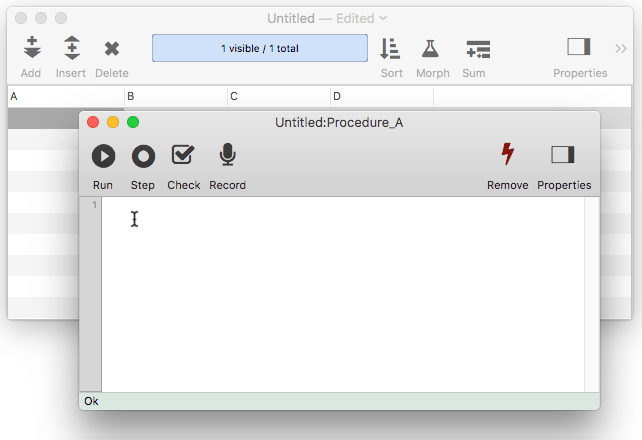This location is usually fairly close to where the actual error is. However, in some cases Panorama is unable to determine exactly where the problem is. Consider the formula shown below, which has a missing left parenthesis. When I press the Check Procedure tool, Panorama indicates that there is a Missing Left Parenthesis and indicates that the error is just before the right parenthesis.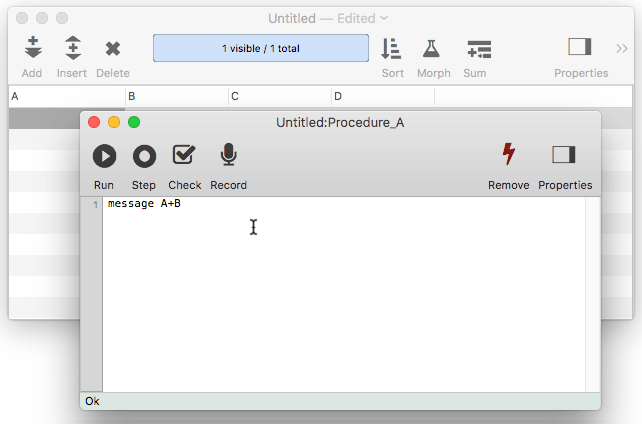But wait — is this really where the error is? No, the error actually is somewhere earlier in the formula. In this case the missing ( probably goes in front of the B or the C. Panorama has done the best job it could to locate the error for you. One thing you do know for sure, though, is that the error is always before the insertion point and not after.

#### Comments

Panorama allows “comments” to be placed inside a formula. A comment is a note within the formula that is ignored when the formula is evaluated. A comment must start with `/*` and end with `*/`. Anything between these will be ignored. (C and JavaScript programmers will recognize this style.) For example, this formula:

``````«C Ratio» /* C Ratio must be updated every 24 hours */ * Amount
``````

will produce the same value as this one:

``````«C Ratio» * Amount
``````

In addition to notes to yourself, comments are also useful for temporarily disabling a section of a long formula without actually deleting the text (for example if you are trying to debug the formula).

See Also

• Arithmetic Formulas -- mathematical operators and functions.
• Characters and Unicode Values -- working with individual characters of text.
• Constants -- values embedded into a formula.
• convertvariablestoconstants -- converts all of the variables in a formula into constant values.
• Date Arithmetic Formulas -- performing calculations on dates, and converting between dates and text.
• Formula Workshop -- formula workshop wizard for testing and experimenting with formulas.
• formulacalc -- allows you to evaluate a formula that you were not able to code into the procedure when it was being written.
• formulafields( -- returns a list of fields used in a formula.
• formulaidentfiers( -- returns a list of identifiers (fields and variables) used in a formula.
• formulavalue -- calculates the result of a formula. Usually this is done with an assignment statement (for example `x=2 * y` ), but the *formulavalue* statement gives you more flexibility. You can specify what database is to be used for the calculation (an assignment statement always uses the current database) and you can specify the formula using a variable, making it easy to change on the fly. This statement also gives you more control over how errors are handled.
• formulavariables( -- returns a list of variables used in a formula.
• Functions -- index of all functions available for use in Panorama formulas.
• Linking with Another Database -- techniques for relating multiple database files so that they work together.
• makemergeformula( -- builds a formula from an “auto-wrap" style merge template.
• Non Decimal Numbers -- working with numbers in alternative (non-decimal) bases, including binary, octal and hexadecimal.
• Numbers -- Working with numeric values in a formula, and converting between numbers and text.
• Operators -- index of all operators available for use in Panorama formulas.
• Quotes -- text constants embedded into a formula
• Statements -- index of all statements available for use in Panorama procedures.
• SuperDates -- date and time combined into a single value.
• Tag Parsing -- Panorama functions for working with text that contains data delimited by tags, including HTML.
• Text Formulas -- manipulating text with a formula (concatenation, extraction, rearranging, etc.)
• Time Arithmetic Formulas -- performing calculations on times, and converting between times and text.
• True/False Formulas -- logical boolean calculations.
• Using Fields in a Formula -- accessing database fields within a formula.
• Values -- the raw material that formulas work with -- numbers and text.
• Variables -- storing and retrieving individual items of data, not part of a database.

History

 Version Status Notes 10.0 No Change Carried over from Panorama 6.0